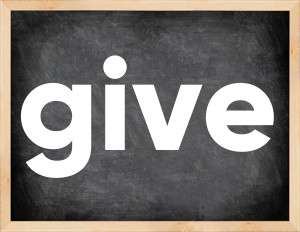# Give past tenseThe English verb 'give' is pronounced as [gɪv].
Related to: irregular verbs.
3 forms of verb give: Infinitive (give), Past Simple - (gave), Past Participle - (given).

## Here are the past tense forms of the verb give

👉 Forms of verb give in future and past simple and past participle.
❓ What is the past tense of give.

## Give: Past, Present, and Participle Forms

Base Form Past Simple Past Participle
give [gɪv]

gave [geɪv]

given [gɪvn]

## What are the 2nd and 3rd forms of the verb give?

🎓 What are the past simple, future simple, present perfect, past perfect, and future perfect forms of the base form (infinitive) 'give'?

### Learn the three forms of the English verb 'give'

• the first form (V1) is 'give' used in present simple and future simple tenses.
• the second form (V2) is 'gave' used in past simple tense.
• the third form (V3) is 'given' used in present perfect and past perfect tenses.

## What are the past tense and past participle of give?

The past tense and past participle of give are: give in past simple is gave, and past participle is given.

### What is the past tense of give?

The past tense of the verb "give" is "gave", and the past participle is "given".

### Verb Tenses

Past simple — give in past simple gave (V2).
Future simple — give in future simple is give (will + V1).
Present Perfect — give in present perfect tense is given (have/has + V3).
Past Perfect — give in past perfect tense is given (had + V3).

### give regular or irregular verb?

👉 Is 'give' a regular or irregular verb? The verb 'give' is irregular verb.

## Examples of Verb give in Sentences

•   Give me a kiss (Present Simple)
•   She gave him her name (Past Simple)
•   His nerves began to give (Past Simple)
•   Please give examples of any relevant action taken (Present Simple)
•   He has given me money, but I'm still not happy (Present Perfect)
•   We are giving serious consideration to this procedure (Present Continuous)
•   My uncle is giving piano lessons! (Present Continuous)
•   Your parents are giving you everything you asked for (Present Continuous)
•   I will not give her another chance (Future Simple)
•   My teacher will give me some money to buy the books (Future Simple)

Along with give, words are popular wash and set.

Verbs by letter: , , , , , , , , , , , , , , , , , , , , , , , , .